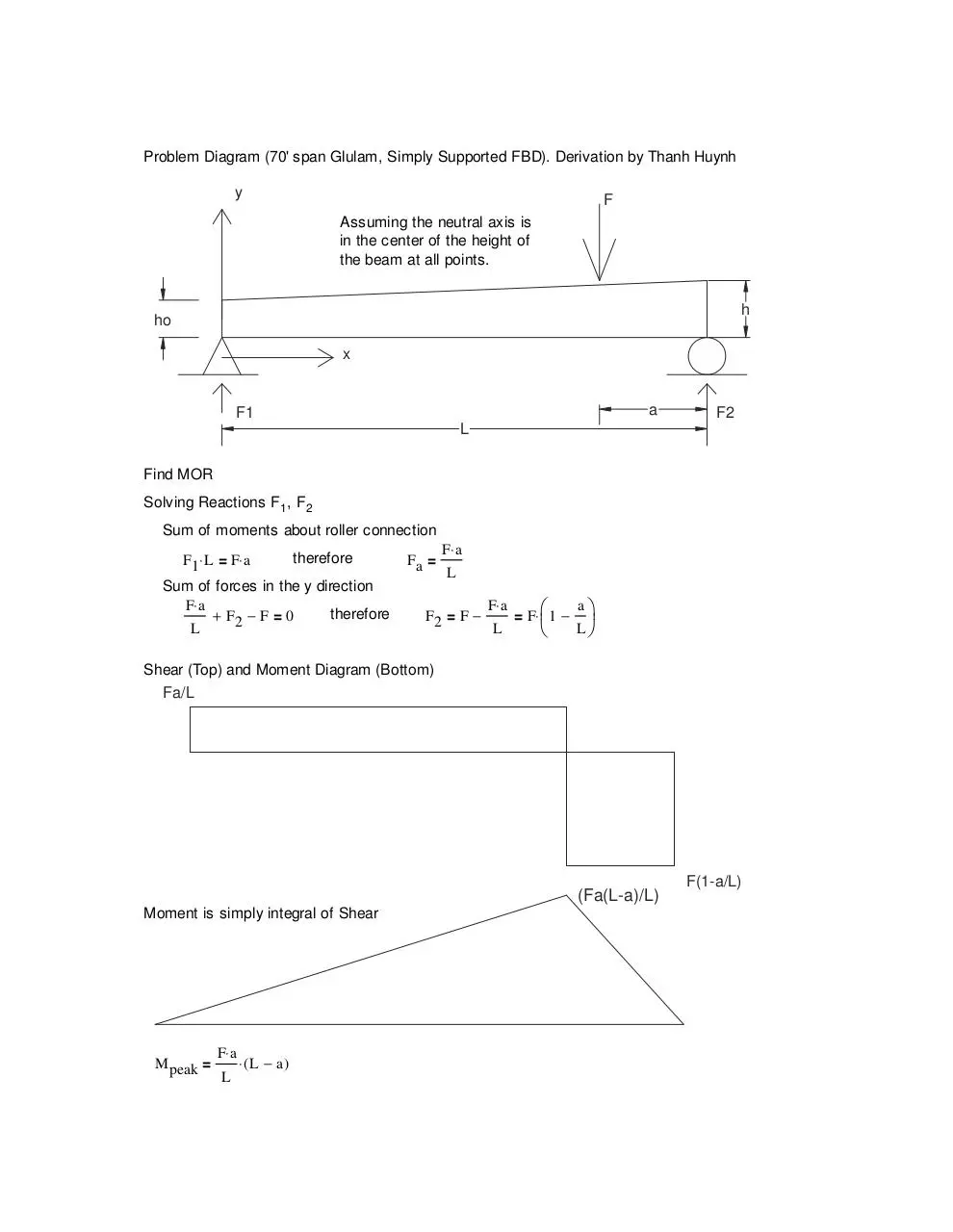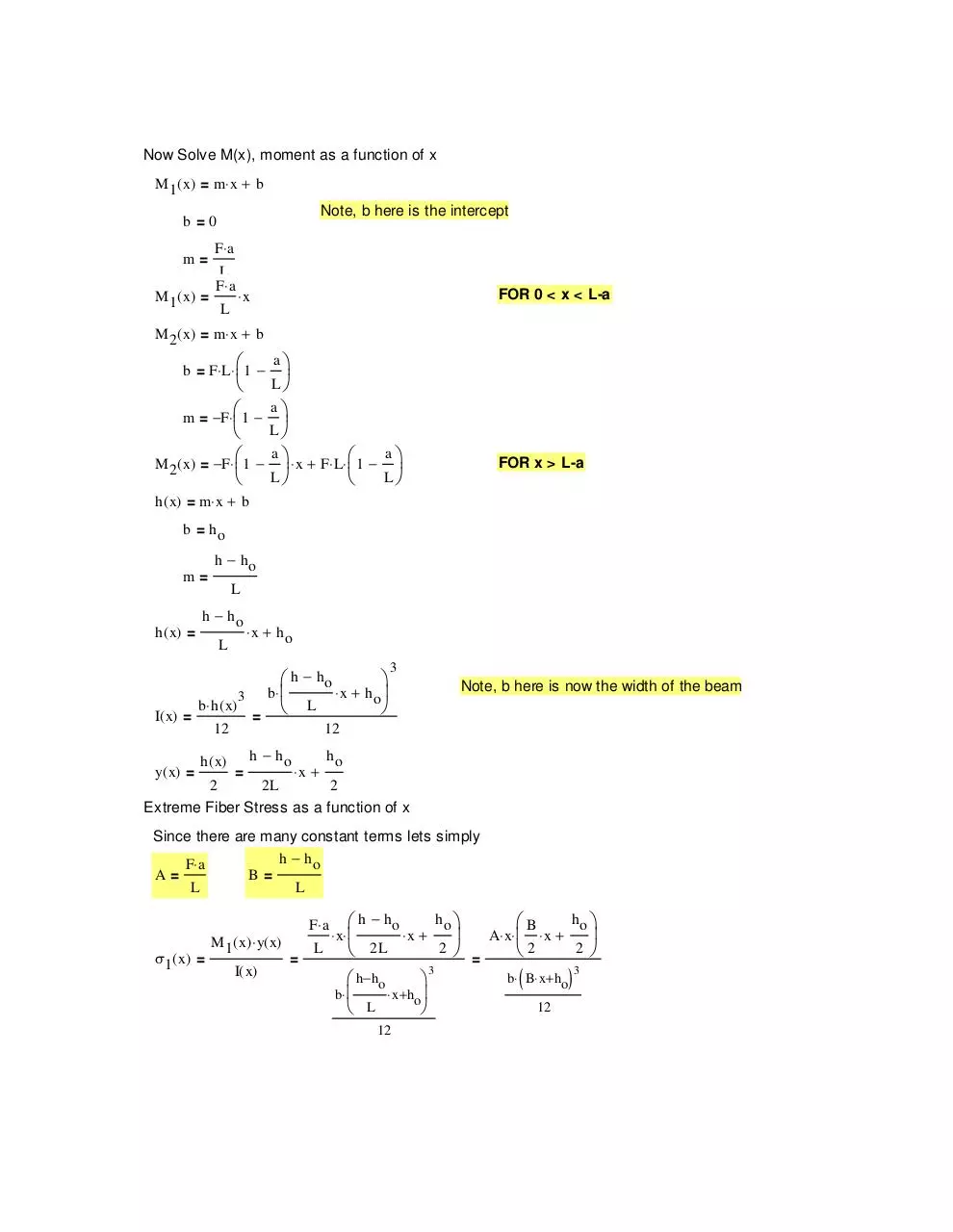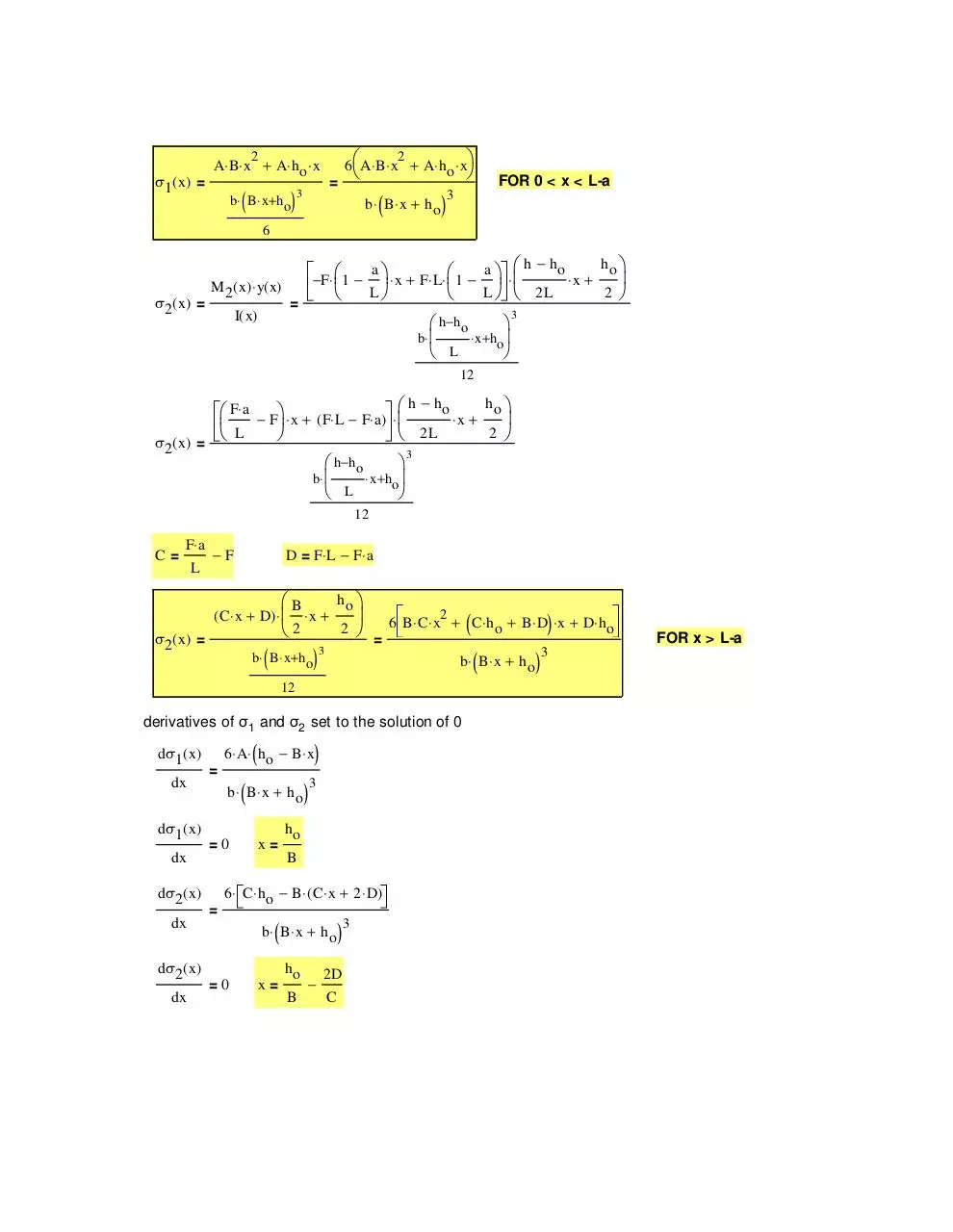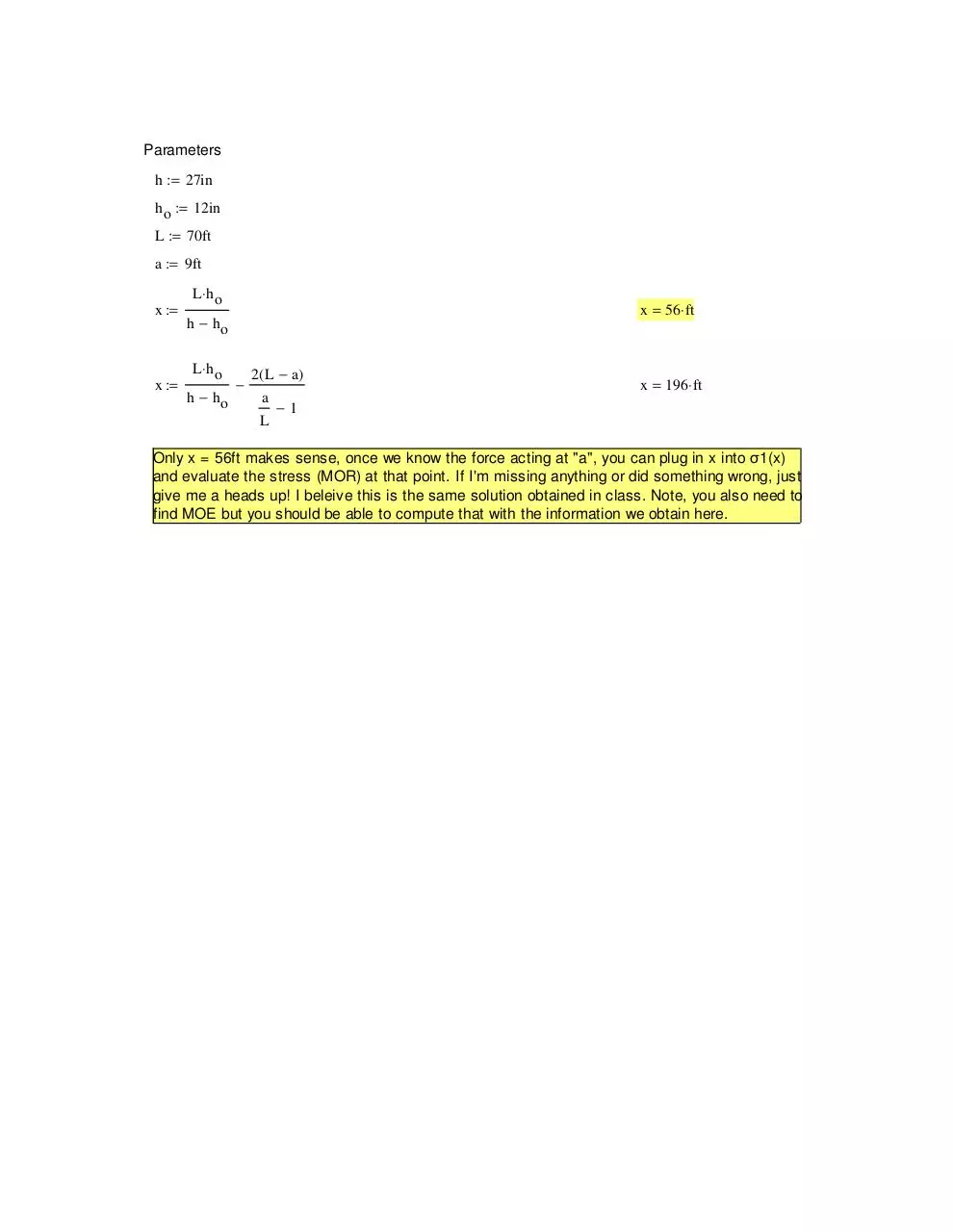### File information

Author: Thanh Q. Huynh

This PDF 1.4 document has been generated by PScript5.dll Version 5.2.2 / GPL Ghostscript 8.71, and has been sent on pdf-archive.com on 31/05/2014 at 03:45, from IP address 50.188.x.x. The current document download page has been viewed 592 times.
File size: 40.65 KB (4 pages).
Privacy: public file

### Document preview

Problem Diagram (70' span Glulam, Simply Supported FBD). Derivation by Thanh Huynh

y

F
Assuming the neutral axis is
in the center of the height of
the beam at all points.

h

ho
x

a

F1

F2

L
Find MOR
Solving Reactions F1 , F2
Sum of moments about roller connection
therefore
F1 ⋅ L = F⋅ a
Sum of forces in the y direction
F⋅ a
therefore
+ F2 − F = 0
L

F⋅ a
Fa =
L
F⋅ a
a
F2 = F −
= F⋅  1 − 
L
L

Shear (Top) and Moment Diagram (Bottom)
Fa/L

(Fa(L-a)/L)
Moment is simply integral of Shear

M peak =

F⋅ a
L

⋅ ( L − a)

F(1-a/L)

Now Solve M(x), moment as a function of x
M 1 ( x) = m⋅ x + b
Note, b here is the intercept

b =0
m=
M 1 ( x) =

F⋅ a
L
F⋅ a
L

FOR 0 &lt; x &lt; L-a

⋅x

M 2 ( x) = m⋅ x + b
b = F⋅ L⋅  1 −

m = −F⋅  1 −

M 2 ( x) = −F⋅  1 −

a

L
a

L

 ⋅ x + F⋅ L⋅  1 −
L

a

a

FOR x &gt; L-a

L

h ( x) = m⋅ x + b
b = ho
m=

h ( x) =

h − ho
L

h − ho
L

⋅ x + ho

 h − ho

b⋅ 
⋅ x + h o
3
b ⋅ h ( x)
 L

I( x) =
=
12

y( x) =

h ( x)

3

Note, b here is now the width of the beam

12

=

h − ho

2

2L

⋅x +

ho
2

Extreme Fiber Stress as a function of x
Since there are many constant terms lets simply
h − ho
F⋅ a
A=
B=
L
L
F⋅ a
σ1 ( x) =

M 1 ( x) ⋅ y( x)
I( x)

=

L

 h − ho

⋅ x⋅ 

 2L

 h− ho

b⋅ 
⋅ x+ho
 L

12

ho 

ho 
B
 A⋅ x⋅  ⋅ x + 
2 
2 
2
=

⋅x +
3

(

b⋅ B⋅ x+ ho
12

)3

2

σ1 ( x) =

(

)

b ⋅ B⋅ x + h o

6  A⋅ B⋅ x + A⋅ h o ⋅ x

2

A⋅ B⋅ x + A⋅ h o ⋅ x

=

3

(

b⋅ B⋅ x + ho

)

FOR 0 &lt; x &lt; L-a

3

6

σ2 ( x) =

−F⋅  1 −
 
 
=

M 2 ( x) ⋅ y( x)

a

 ⋅ x + F⋅ L⋅  1 −
L

I( x)

ho 
 h − ho
⋅x +

 ⋅ 
L   2L
2 
a 

 h− ho
b⋅ 
⋅ x+ ho
 L

3

12

h
 F⋅ a − F ⋅ x + ( F⋅ L − F⋅ a) ⋅  h − h o ⋅ x + o 
 L

2 


  2L
σ2 ( x) =

 h− ho

b⋅ 
⋅ x+ ho
 L

3

12

C=

F⋅ a
L

D = F⋅ L − F⋅ a

−F

ho 
B
⋅x +
 6 B⋅ C⋅ x2 + C⋅ h + B⋅ D ⋅ x + D⋅ h 
( o
)
2 
o
2

=

( C⋅ x + D) ⋅ 
σ2 ( x) =

(

)

b⋅ B⋅ x+ ho

3

(

b⋅ B⋅ x + h o

12

derivatives of σ1 and σ2 set to the solution of 0
dσ1 ( x)

=

dx
dσ1 ( x)
dx
dσ2 ( x)

(

(

b ⋅ B⋅ x + ho
=0

=

dx

x=

)

3

ho
B

6 ⋅ C⋅ h o − B⋅ ( C⋅ x + 2 ⋅ D)

dx
dσ2 ( x)

)

6 ⋅ A⋅ h o − B⋅ x

(

b ⋅ B⋅ x + h o
=0

x=

ho
B

)

2D
C

3

)

3

FOR x &gt; L-a

Parameters
h := 27in
h o := 12in
L := 70ft
a := 9ft
x :=

x :=

L⋅ h o

x = 56⋅ ft

h − ho
L⋅ h o
h − ho

2 ( L − a)
a
L

x = 196 ⋅ ft

−1

Only x = 56ft makes sense, once we know the force acting at "a", you can plug in x into σ1(x)
and evaluate the stress (MOR) at that point. If I'm missing anything or did something wrong, just
give me a heads up! I beleive this is the same solution obtained in class. Note, you also need to
find MOE but you should be able to compute that with the information we obtain here.Mathcad - Lab7 - Derivation.pdf (PDF, 40.65 KB)

### Share on social networks

#### HTML Code

Copy the following HTML code to share your document on a Website or Blog